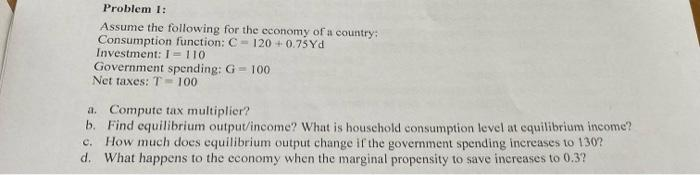Home / Expert Answers / Economics / problem-1-assume-the-following-for-the-economy-of-a-country-consumption-function-c-120-0-75yd-pa824

# (Solved): Problem 1: Assume the following for the economy of a country: Consumption function: C = 120 +0.75Yd ...

Problem 1: Assume the following for the economy of a country: Consumption function: C = 120 +0.75Yd Investment: I = 110 Government spending: G = 100 Net taxes: T = 100 a. Compute tax multiplier? b. Find equilibrium output/income? What is household consumption level at equilibrium income? How much does equilibrium output change if the government spending increases to 130? d. What happens to the economy when the marginal propensity to save increases to 0.3? C.Problem 1: Assume the following for the cconomy of a country; Consumption function: Investment: Government spending: Net taxes: a. Compute tax multiplier? b. Find equilibrium output/income? What is household consumption level at equilibrium income? c. How much does equilibrium output change if the government spending inereases to 130? d. What happens to the economy when the marginal propensity to save inereases to 0.3 ?

We have an Answer from Expert

a. The tax multiplier can be calculated using the formula: Tax Multiplier = -MPC / (1 - MPC), where MPC is the marginal propensity to consume.
Given that net taxes (T) are equal to 100 and the consumption function is C = 120 + 0.75Yd, we can infer that Yd (disposable income) is equal to Y (output/income) minus taxes (T): Yd = Y - T.
The marginal propensity to consume (MPC) is the change in consumption divided by the change in disposable income: MPC = ?C / ?Yd.
In this case, since net taxes (T) are constant at 100, there is no change in taxes. Therefore, the change in consumption (?C) is equal to the change in disposable income (?Yd). Hence, MPC = ?C / ?Yd = (C - C?) / (Yd - Yd?) = (C - C?) / (Y - Y? - T), where C? and Yd? represent the initial consumption and disposable income, respectively.
Plugging in the values from the given consumption function: C = 120 + 0.75Yd, we can rewrite it as Yd = C - 120, which gives us the expression for MPC: MPC = (C - C?) / (Y - Y? - T) = (C - C?) / (Y - Y? - 100).
Since there is no information provided about the initial consumption (C?), we cannot directly calculate MPC. Therefore, we cannot determine the tax multiplier without this information.